# Pearson's Product Moment Correlation

The correlation between two variables,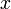$x$ and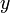$y$, with weighted means of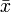$\bar{x}$ and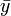$\bar{y}$ respectively, is: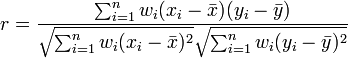$r = \frac{\sum ^n _{i=1}w_i(x_i - \bar{x})(y_i - \bar{y})}{\sqrt{\sum ^n _{i=1}w_i(x_i - \bar{x})^2} \sqrt{\sum ^n _{i=1}w_i(y_i - \bar{y})^2}}$$w_i$ is the Calibrated Weight for the$i$th of$n$ observations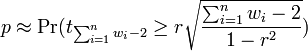$p \approx \Pr(t_{\sum^n_{i=1}w_i-2} \ge r\sqrt{\frac{\sum^n_{i=1}w_i-2}{1 - r^2}})$